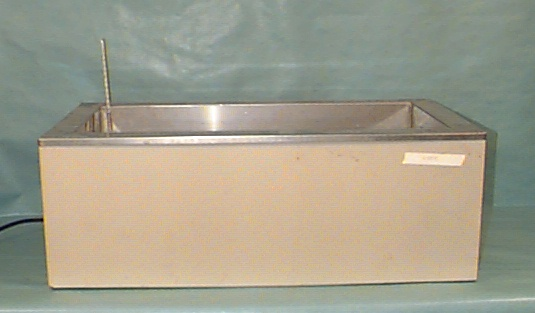## Laboratory Procedure

Work with 2 partners; each partner will conduct the experiment at a different temperature, then share data with the group.

I. Pre-lab Data

MW aspirin (ASA) = _________________

MW salicylic acid = _______________

1 mg salicylic acid represents ____________ mg ASA degraded

II. Experimental Data and Results

(Show calculations and include all units)

A. Standard Curve

Determine the UV absorbance of salicylic acid standards at 310 nm using UV grade cuvettes. Use water as a blank. Construct a Beer’s Law plot of absorbance vs. concentration. Determine the slope, intercept, and R2 value. Remember, [0,0] is a valid point.

A 2.0 mg/ml stock solution of salicylic acid will be supplied. Follow the dilution scheme to make a series of salicylic acid standards as indicated in test tubes. Use water as the diluent.

To make a Beer’s Law plot for today’s experiment, take 1 ml of the salicylic acid standards and add 14 mls of water, and read with a spectrophotometer at 310 nm. Such a dilution will result in the following concentrations:

2. Hydrolysis of Aspirin (ASA)

1. Place approximately 120 ml of buffer solution (0.067 M phosphate buffer, pH = 7.5) into a covered flask and put the flask into the constant temperature bath. Allow the contents to come to the specified temperature (about 20 – 30 minutes).1. Weigh exactly 200 mg of aspirin and transfer the powder to a 100 ml volumetric flasks. Add 2.0 ml of Alcohol, USP to dissolve the aspirin, and q.s. to 100 ml with hot buffer solution. Mix this solution well by inverting the covered volumetric flask several times. Immediately pipet a 1 ml sample and place the volumetric flask in the water bath. Empty the contents of the pipet into a test tube. This sample allows the determination of the amount of aspirin which has already hydrolyzed by the time the flask is placed in the water bath. This sample will correspond to time zero.
2. Add 14 ml of distilled water to the 1.0 ml sample in the test tube and mix well by inverting the covered test tube several times. Read the absorbance of the solution at 310 nm. A blank of distilled water is also needed.
3. Continue to pipet and analyze 1 ml samples from the buffer solution in the water bath every 15 minutes for 75 minutes. Do not remove the flask from the bath when sampling so that temperature can remain stable.
4. Determine the concentration of salicylic acid (SA) in each sample using values from your Beer’s Law plot, and the following equation rearrangement:5. Calculate the amount (in mg) of ASA degraded at each sample time (mg ASA degraded = Conc SA in mg/ml × Dilution Factor × 100 ml × 1.30)

6. Calculate the amount of ASA remaining at each sample time (mg ASA remaining = initial wt. ASA – wt. ASA degraded)

Temperature 1 =_____

Time Abs Conc of SA (mg/ml) ASA degraded (mg) ASA remaining (mg)
0
15
30
45
60
75

Temperature 2 =______

Time Abs Conc of SA (mg/ml) ASA degraded (mg) ASA remaining (mg)
0
15
30
45
60
75

Temperature 3 = _____

Time Abs Conc of SA (mg/ml) ASA degraded (mg) ASA remaining (mg)
0
15
30
45
60
75

III. Calculation of Kapp and K at Elevated Temperatures:

1. Plot ln ASA remaining vs. time for each temperature.

2. Determine the slope of the line at each temperature.

3. Calculate Kapp at each temperature: -Kapp = slope

4. Calculate K for each temperature: K = Kapp/[OH]

Temp (°C) Temp (°K) 1/T°K Kapp (min-1) K (min-1)

IV. Determination of Kapp and K at Room Temperature.

Construct an Arrhenius plot.

• Plot ln Kapp vs. 1/T (°K) and determine the slope and the activation energy for the hydrolysis of ASA. (Ea = -slope × R)
• Determine the Kapp and K at room temperature.

From Arrenhius plot:

Slope ______________ Ea ________________

At room temperature:

Kapp __________________ K ___________________